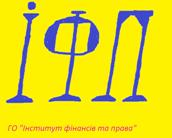PUBLIC ORGANIZATION «INSTITUTE OF FINANCE AND LAW»

vul. Shevchenka 27, . 36, Poltava , UKRAINE, tel (067) 401-93-74, fax: (0532) 67-73-38, www.institutefl.org, ел. пошта: institute_fl@institutefl.org

1) The official currency exchange rate of the Ukrainian Hryvnya to the foreign currencies:

2) The interbank exchange rate of the Ukrainian Hryvnya to the foreign currencies:

ii

2) The cash currency exchange rate of the Ukrainian Hryvnya to the foreign currencies:

© Public Organization Institute of Finance and Law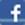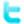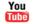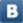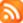Ęóđń Âŕëţň Číôîđěĺđ Óęđŕčíńęčő ăđčâĺí(AZN) //-//(AMD) //-//(MDL) //-//(KZT) //-//(KGS) //-//(RUB) //-//(TJS) //-//(UZS) //-//(BYR) //-//(CZK) //-//(DKK) //-//(HUF) //-//(ISK) //-//(LVL) //-//(LTL) //-//(NOK) //-//(RON) //-//(SEK) //-//(CHF) //-//(TRY) //-//(GBP) //-//(BGN) //-//(EUR) //-//(PLN) //-//(CAD) //-//(CNY) //-//(USD) //-//(BRL) //-//(INR) //-//(JPY) //-//(KRW) //-//(KWD) //-//(SGD) //-//(ZAR) //-//(AUD) //-//